Get instant live expert help with Excel or Google Sheets“My Excelchat expert helped me in less than 20 minutes, saving me what would have been 5 hours of work!”

#### Post your problem and you’ll get Expert help in seconds.

Your message must be at least 40 characters
Our professional Expert are available now. Your privacy is guaranteed.

# Remove Line Breaks in Excel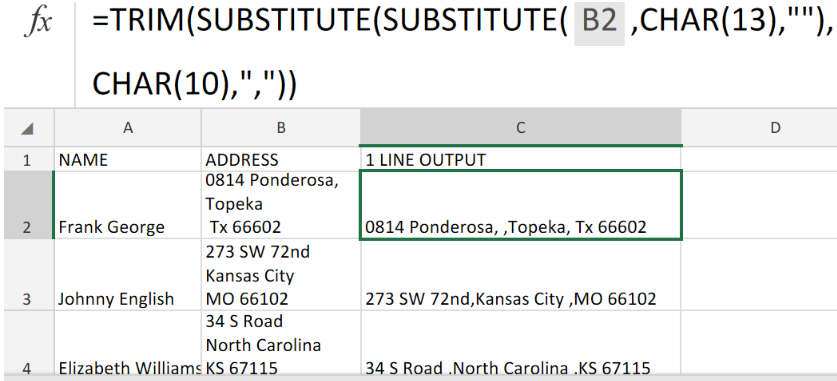Figure 1. of Remove Line Breaks in Excel.

In order to remove any/all Line Breaks from text located within a cell of our worksheet, we are going to utilize a formula syntax based on the CHAR and SUBSTITUTE Functions in Excel.

## Generic Formula

`=SUBSTITUTE(A1,CHAR(10),", ")`

## How to Remove Line Breaks in Excel

There are 3 different and simple ways to remove Line from text in Excel.

1. Removing Line Breaks by using the SUBSTITUTE Function.

We are going to make use of the SUBSTITUTE Function which is able to locate matching text inside any cell and then replace such with any specific text of your choosing.

In the illustration below, we are going to remove any Line Breaks from our text and replace them all with commas.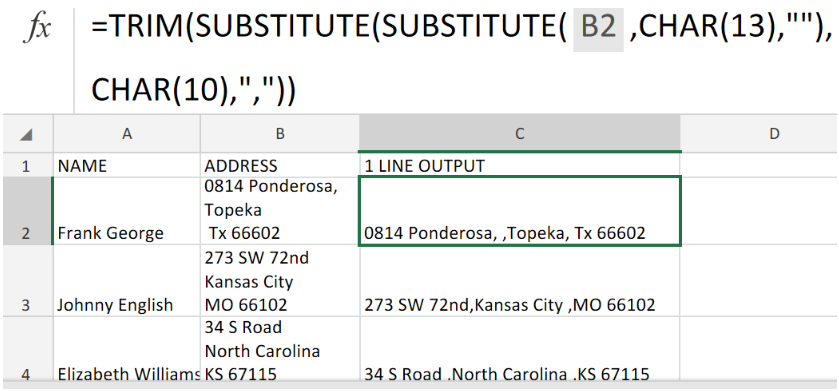Figure 2. of Substitute Function in Excel.

The formula syntax which we have assigned to cell C2, in the example above, is as follows;

`=TRIM(SUBSTITUTE(SUBSTITUTE(B2,CHAR(13),""),CHAR(10),","))`

This will automatically remove any unwanted line Breaks, replace them with commas and give us the results displayed in the 1 LINE OUTPUT column of our worksheet illustration.

2. Removing Line Breaks by using the CLEAN Function.

The Excel CLEAN Function operates by taking a text string and returning it free from any line breaks and other unwanted characters.

### Generic Formula

`=CLEAN(text)`

• We enter the following formula in cell C3 `=CLEAN(B3)`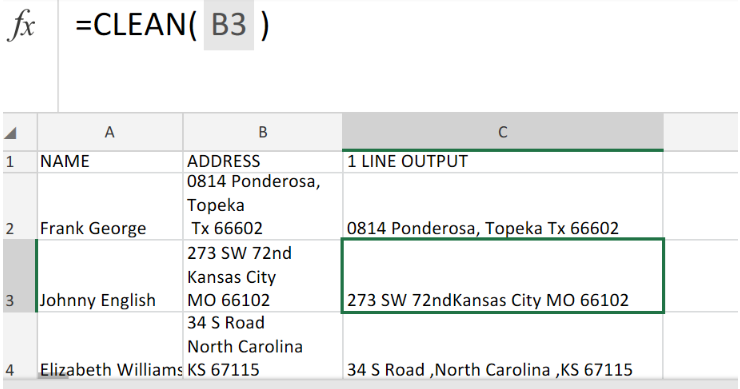Figure 3. of Clean Function in Excel.

This will automatically remove any unwanted characters from the text in column B of our worksheet.

3. Removing Line Breaks by using the TRIM Function.

The Excel TRIM Function operates in the same manner as the CLEAN Function.

### Generic Formula

`=TRIM(text)`

• We enter the following formula in cell C4 `=TRIM(B4)`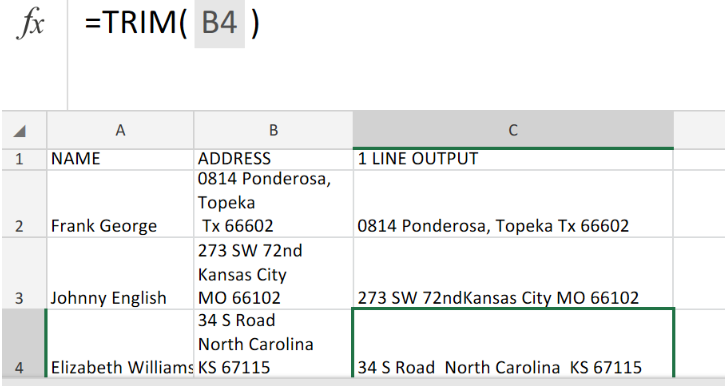Figure 4. of Trim Function in Excel.

This will automatically remove any unwanted spaces or line breaks from the text in column B.

In conclusion, Excel has three built-in Functions – SUBSTITUTE, TRIM and CLEAN – that are designed to automatically remove any extra space and line breaks from text.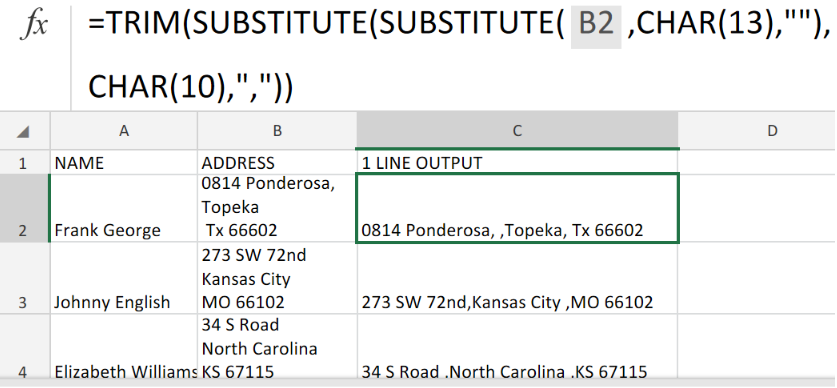Figure 5. of Final Result.

## Instant Connection to an Expert through our Excelchat Service

Our live Excelchat Service is here for you. We have Excel Experts available 24/7 to answer any Excel questions you may have. Guaranteed connection within 30 seconds and a customized solution for you within 20 minutes

### Did this post not answer your question? Get a solution from connecting with the expert.Another blog reader asked this question today on Excelchat:
Solution examplesI am trying to figure out how to use a sumif on SUBSTITUTE(A2,"*","~*") where A2 is a formula with 3371-4
Solved by M. Y. in 19 minsHello, I need help with IF and SUBSTITUTE formula in excel
Solved by A. A. in 43 minsI have 4 column. when i fill the first 3 column. in 4th column i need a unique id number and i generate unique id number using RANDBETWEEN formula [ =CHAR(RANDBETWEEN(65,90))&CHAR(RANDBETWEEN(65,90))&RANDBETWEEN(1000,9900) ] but i want ..... when i'm filling the 3 column after that 4th column run this formula and generate unique id automatically
Solved by X. Q. in 20 minswhen i fill column A and B then C column generate Unique ID using RandBetween Formula {=CHAR(RANDBETWEEN(65,90))&CHAR(RANDBETWEEN(65,90))&RANDBETWEEN(1000,9900)} ...
Solved by S. Q. in 20 mins## Subscribe to Excelchat.coAnother blog reader asked this question today on Excelchat: